# Absolutely central elastic collision (or translate lying)

• Gustavs1337
In summary, the two balls have the same size but different masses, and after an elastic collision they each have a different speed.f

## Homework Statement

2 balls, their size is the same but mass is different, they are going towards a collision. I do not know what is the word for it in english but its like this m1= 1,2m2. Speed of the balls are v1=12m/s and v2= 15m/s.Calculate the speed of each ball after absolutely central elastic collision.

p=mv
p=p,

## The Attempt at a Solution

I could not even think of a solution since I missed out some classes.

What's conserved in an elastic collision?

I don't see the connection between the relevant equations and the attempt at solution ?

What's conserved in an elastic collision?

I don't see the connection between the relevant equations and the attempt at solution ?
yes all i can write is p1= 1,2m2 x 12 = 14,4m2 but i can't get past this part, the p= p, is just a reminder for me. And i think that its kinetic energy that's being conserved.

the p= p, is just a reminder for me.
It should remind you that momentum is conserved.

And i think that its kinetic energy that's being conserved.
Right.

So combine those two conservation laws.

Its going to be hard since english is not my born language. so i couldn't figure it out so i looked on the internet and this is what i got K = 1/2m(p/m)2= p2/2m

Its going to be hard since english is not my born language. so i couldn't figure it out so i looked on the internet and this is what i got K = 1/2m(p/m)2= p2/2m
That's just expressing the kinetic energy in terms of momentum instead of velocity. That won't help you.

You need to write equations for:
• conservation of energy
• conservation of momentum
I assume you have a textbook. I suspect it gives examples of this sort of collision.

As I said before I missed out on some classes, they were doing the similar but easier tasks and I did not ask for their notes so that is my fault. Conservation of momentum is m1xdv1=-m2xdv2 as it says. And Energy i don't know that, or maybe its because of the differences in language.

Conservation of momentum is m1xdv1=-m2xdv2 as it says.
There are four velocities involved: The initial and final velocities of each object.

And Energy i don't know that, or maybe its because of the differences in language.
I don't think language has much to do with it.And read this: Wiki: Elastic Collisions in one dimension

There are four velocities involved: The initial and final velocities of each object.
so you mean i should do it like this ?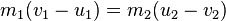or this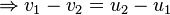? And about that energy, i could not find anything but Ek= Ek'. There is also this ∑p = constant but i doubt it, also Ek= mv2/2 but also i doubt that. Only equation that i think would be right is the first one. :/. I have something to add
m1v1 + m2v2 =[m1+m2]v3 mayb e this

Last edited:
Both conservation equations are given in that Wiki link. They even solve them for you!

Both conservation equations are given in that Wiki link. They even solve them for you!
so basically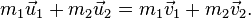this is the momentum equation as it says and this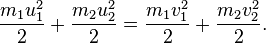is energy equation.
and i get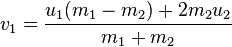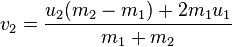by combining them ?

That's correct. (But you'd better understand how to solve those equations.)

You want me to put numbers in those equations or understand how i got the combinations, i have a hard time understanding what comes next.

You want me to put numbers in those equations or understand how i got the combinations, i have a hard time understanding what comes next.
Yes, just plug the numbers in.
By the way, the correct translation is probably "head-on elastic collision", i.e. the mass centres, direction of relative motion and point of impact are all in a straight line.